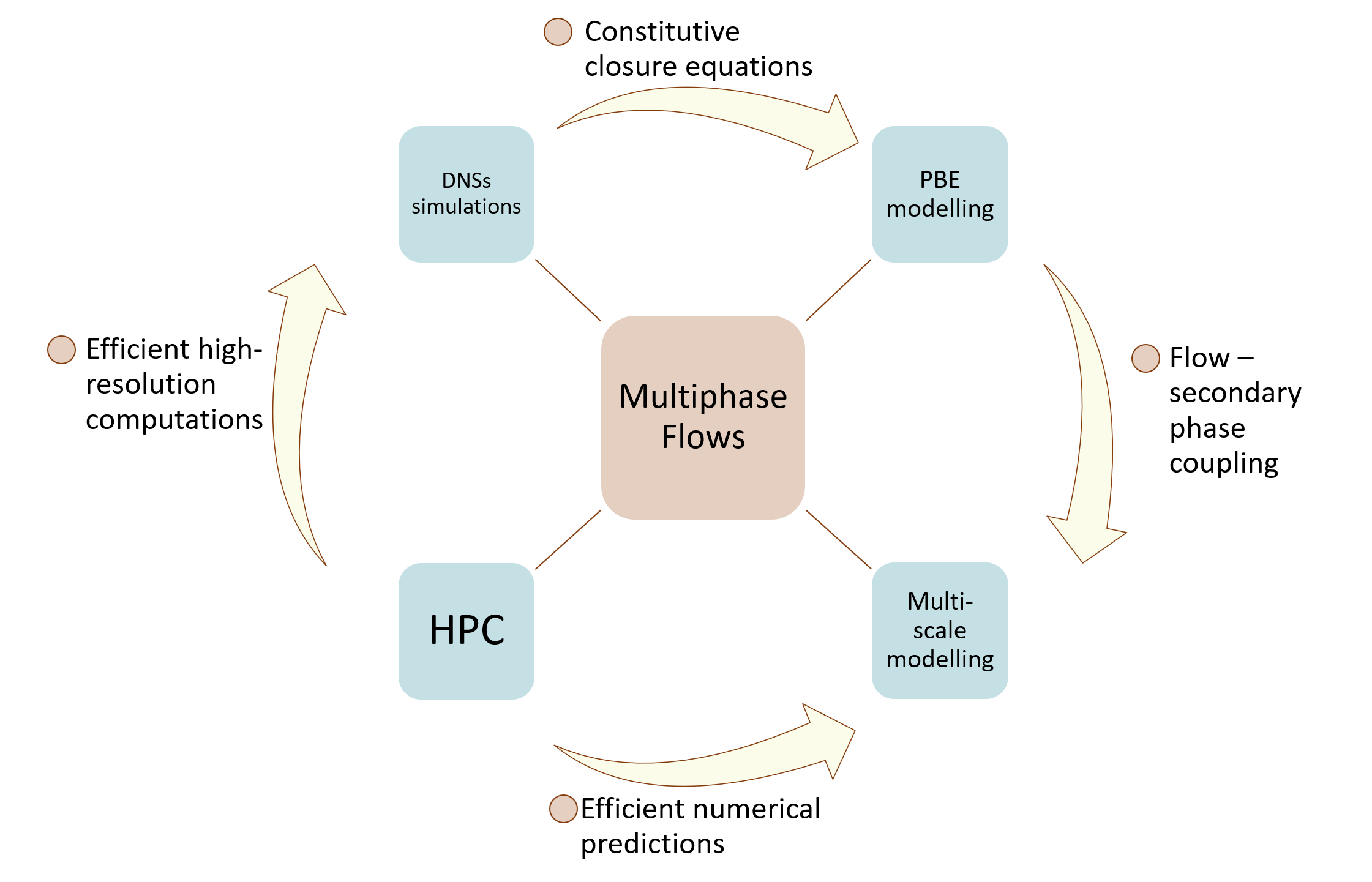﻿ Home

# Welcome to NM2F Group

Welcome to the Numerical Modelling of Multiphase Flows Research Group at the Department of Mechanical Engineering at the University of Alberta. This Group is led by Associate Professor Alexandra Komrakova.

# Research InterestsMultiphase systems contain at least two distinct phases such as gas/vapour, liquid or solid. From a broad diversity of these systems, we focus on those where liquid or gas acts as a continuous (carrier) phase and the second phase being either gas, liquid, or solid represents the dispersed phase (bubbles, drops, and particles). The fascinating research questions about multiphase systems are related to the interaction between the phases. How will turbulent flow change if we introduce particles or drops into it? Or if we expose liquid-liquid dispersion to vigorous agitation, what distribution of drop sizes will we get? To answer these questions, we need comprehensive understanding of hydrodynamics of the flow and interfacial phenomena.

In our group, we develop numerical models to seek an in-depth understanding of multiphase flows. The models that we create, differ depending on the application. A thorough investigation of complex liquid-liquid interface behaviour in turbulent flow conditions requires sophisticated and computationally expensive direct numerical simulations (DNSs). Time- and resource-demanding computations produce terabytes of data that we process, understand, and turn into useful knowledge. The generated fundamental knowledge is converted to simpler closure relationships and constitutive equations that we can use in multiscale modeling and population balance equations (PBEs). Computational fluid dynamics (CFD) based models are used to study complex flow behaviour on bench and lab-scale when DNSs are unaffordable.

Numerical modelling is a powerful research tool that is used for meaningful visualization and quantification of complex flows. With current computational facilities, we can dynamically resolve length scales of less than a micron and time scales less than a millisecond. We can quantify physical properties of the system that cannot be directly measured experimentally, for instance interfacial energy of high deformed interface.

The key aspects of our research direction are
• Development and application of numerical models for direct numerical simulations (DNSs) of multiphase flows
• High performance computing (HPC)
• Development and application of population balance equation (PBE) models
• Multiscale CFD simulations of complex multiphase flows
• Cross-validation of numerical models